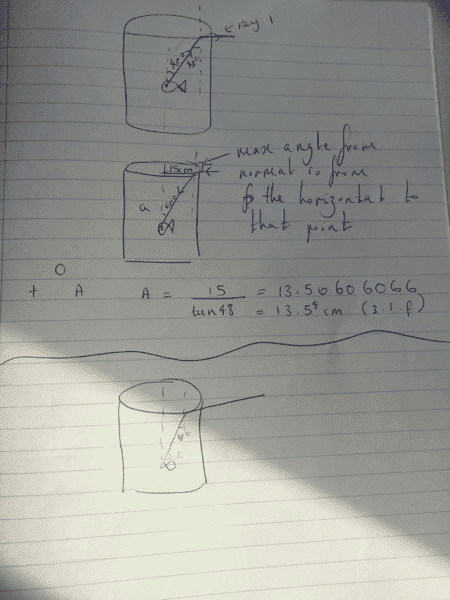# Critical angle: find the depth of the fish looking up

## Homework Statement

A fish floats in water with its eye at the centre of an opaque walled full tank of water of circular cross section. When the fish look upwards, it can see a fish-eye view of the surrounding scene i.e. it is able to view the hemisphere of the scene above the water surface, and centred at the top of the tank. The diameter of the tank is 30 cm, and the critical angle for water is 48 degrees. At what depth below the surface of the water, d, must the fish be floating?
A) 16.7cm
B) 13.5cm
C) 11.2cm
D) 10.0cm

## The Attempt at a Solution

From the question you can tell that okay, I'm going to have to use the critical angle and since the fish's eyes are in the center we will get a right angle triangle with a side of 15 cm and an angle with 48 degrees from there I can use trigonometry to work out the side. Since I want the vertical I would have ended up with two answers
10.0cm and 13.5cm. The actual answer is 13.5cm.
Now I don't understand the questions. The mark scheme says "From the fishes eye to the rim of the tank makes an angle of 48o with the vertical. The emerging ray is horizontal (which is the requirement to see the horizon). Hence the depth of the fish is, d x tan 48o = 15.0, giving d."
"it is able to view the hemisphere of the scene above the water surface, and centered at the top of the tank." What does this mean? what does the hemisphere of the scene mean and centers at the top of the tank? If it is from above the water's surface, then would it just refract critically at the surface of the water and not reach the fish's eyes?

#### Attachments

•28.2 KB · Views: 366

RPinPA
Homework Helper
"it is able to view the hemisphere of the scene above the water surface, and centered at the top of the tank." What does this mean?

Think of the "bowl of the sky", what the ancients thought was an actual sphere around us. The fish can see anything that is at the level of the surface or higher.

If it is from above the water's surface, then would it just refract critically at the surface of the water and not reach the fish's eyes?
If it refracts, that means it penetrates the water and therefore could reach something in the water like the fish. What happens at the critical angle is that a ray going from water to air is refracted at 90 degrees, just along the surface. So in the other direction a ray along the surface will be refracted at the critical angle into the water.

The rays farthest from the normal, the rays with the largest angle, are the ones that go to the rim of the tank. So if the line from the fish to the surface at the rim is at the critical angle, he'll be able to see rays coming from the horizontal to that point. If he looks up at the surface at any other place inside the tank, the angle is less than the critical angle and the incident rays he sees will be at angles above the horizontal.

So the place that determines the limits of his vision is at the rim of the water, where the water meets the edge of the tank.

Does the explanation make sense to you now?

jbriggs444
Homework Helper
"it is able to view the hemisphere of the scene above the water surface, and centered at the top of the tank." What does this mean?
In my opinion, the question is garbage. The fish could be one inch below the center of the tank and see the same hemisphere as when it is deeper.

So is that a gap in my knowledge? Is it a fact that a refracted ray lets say ray 1 if there was to be an incident ray that is the same as ray 1 except in the opposite direction will produce a refracted ray that is the same as the incident ray to ray 1. It's indicated in my diagrams if that sentence was confusing.

If he looks up at the surface at any other place inside the tank, the angle is less than the critical angle and the incident rays he sees will be at angles above the horizontal.
So if the fish was above the 13.5 cm the angle would be greater so you would get total internal reflections and if he was below than it would refract out of the container but not at 90 degrees to the container.#### Attachments

In my opinion, the question is garbage. The fish could be one inch below the center of the tank and see the same hemisphere as when it is deeper.

Using trig isn't the only option 13.5 cm